Mobile QR Code1. (Dept. of Electrical Engineering, Pusan National University, Busan 46241, Korea.)
2. (Dept. of Electronic Engineering, Daegu University, Gyeongsan 38453, Korea)

## I. INTRODUCTION

Recently traffic accidents due to driver's physical abnormalities are increasing. In particular, as the number of elderly drivers is increasing as we enter the aging society, research on a biometric monitoring radar system that monitors the driver's abnormal vital signs more accurately while driving to prevent traffic accidents due to the driver's condition abnormality is currently being conducted (1-5). In order to measure the driver's heartbeat or respiration in a non-contact manner, research on a radar sensing transceiver using a millimeter wave (mm-wave) band is in progress. If the millimeter wave band is used, the size of the radar module can be reduced and high resolution characteristics can be obtained.

In order to integrate in a vehicle at low cost, it is necessary to use a direct conversion receiver that can minimize the use of external elements. Since the direct conversion receiver structure is used in the mm-wave band, the balancing characteristic between the I/Q signal paths of the mm-wave receiver is important. Since radar interference signals may exist in the 24 GHz band, a receiver front end with good linearity is required.

Therefore, a 24-GHz receiver front-end is proposed for in-cabin radar systems that can monitor driver’s vital signs such as heart rate and respiration in this paper. It was implemented using a 1-poly 8-metal RF 65-nm CMOS process. In the following sections, the circuit design and measured results of the receiver front-end are described in details.

## II. Proposed Circuit Design

Fig. 1 shows the simplified block diagram of the presented 24 GHz direct-conversion receiver front-end. A direct-conversion receiver architecture is suitable for a wireless short-range monitoring systems in a car because it has high integration and low power consumption. The presented 24 GHz receiver front-end consists of a low-noise amplifier, an I(in-phase)/Q(quadrature) down-conversion mixer, and an I/Q LO generator. The RF input signal from the antenna is applied to the input of the low-noise amplifier and amplified by the low-noise amplifier. The amplified RF signal from the low-noise amplifier is converted into the baseband analog I/Q output signal using the LO input signal, which comes from an external LO signal source or an internal frequency synthesizer, by the I/Q down-conversion mixer.

The simplified schematic of a low-noise amplifier (LNA) is shown in Fig. 2. It is composed of a single-ended cascode amplifier and a single-to-differential common-source amplifier. To improve the electro-static discharge (ESD) protection performance of the LNA, the single-ended cascade amplifier with shunt inductance L$_{\mathrm{g1}}$ at input stage is employed. The input impedance Z$_{\mathrm{in}}$ of the LNA is approximately expressed as

Fig. 1. Block diagram of the proposed receiver front-end.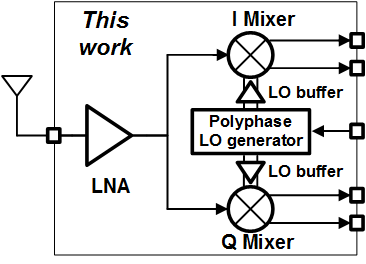##### (1)
\begin{aligned} Z_{\text {in }}(\omega) & =j \omega L_{g 1} \|\left[\frac{g_{m} L_{s 1}}{C_{g s}}+j \omega\left(L_{g 2}+L_{s 1}\right)+\frac{1}{j \omega C_{1}}+\frac{1}{j \omega C_{g s}}\right] \\ & \approx j \omega L_{g 1} \|\left[\frac{g_{m} L_{s 1}}{C_{g s}}+j \omega\left(L_{g 2}+L_{s 1}\right)+\frac{1}{j \omega C_{g s}}\right] \end{aligned}

where g$_{m}$ and C$_{gs}$ are the transconductance and gate-source capacitance of the transistor M$_{1}$, respectively (6). Since the value of the capacitance C$_{1}$ is much larger than that of the capacitance C$_{gs}$, 1/j${\omega}$C$_{1}$ can be ignored in (1). To make the imaginary part of (1) zero, the following (2) must be satisfied at the operating frequency f$_{op}$;

##### (2)
$f_{op}=\frac{1}{2\pi \sqrt{g_{m}^{2}L_{s1}^{2}+C_{gs}(L_{g1}+2L_{g2}+2L_{s1})}}$

When (2) is satisfied, (1) is can be expressed as

##### (3)
$Z_{in}(\omega )\approx \frac{g_{m}C_{gs}L_{s1}^{3}}{(2g_{m}^{2}L_{s1}^{2}- C_{gs}L_{g1})[g_{m}^{2}L_{s1}^{2}+C_{gs}(L_{g1}+2L_{g2}+2L_{s1})]}$.

Therefore, in order to match the input impedance Z$_{\mathrm{in}}$ of the LNA to 50 Ω, the LNA was designed by adjusting the design parameters so that (3)becomes 50 Ω.

As shown in Fig. 3, a test bench was set up for the human body model (HBM) ESD simulation (7). In order to examine the effect of the metal width of the inductance L$_{\mathrm{g1}}$ on the NF and HBM characteristics of the LNA, simulation was performed by changing the metal width of the inductance L$_{\mathrm{g1}}$ under the same inductance condition. Fig. 4 shows the simulated NF and HBM results of the LNA according to the metal width of the inductance L$_{\mathrm{g1}}$. When the metal width of L$_{\mathrm{g1}}$ is 2 µm, the simulated quality factor of L$_{\mathrm{g1}}$ is 18. When the width is 5~µm and 10 µm, the simulated quality factor of the inductor is 19 and 20, respectively. Regardless of the metal width of L$_{\mathrm{g1}}$, the simulated NF of the LNA is approximately 3.2 dB, and the HBM voltage is about 200~V. Considering the NF and HBM performances of the LNA, the metal width of the inductance L$_{\mathrm{g1}}$ was used as 2 µm in our design.

Fig. 4. Simulated human body model(HBM) test result and NF of the LNA according to the metal width of inductor L$_{\mathrm{g1}}$.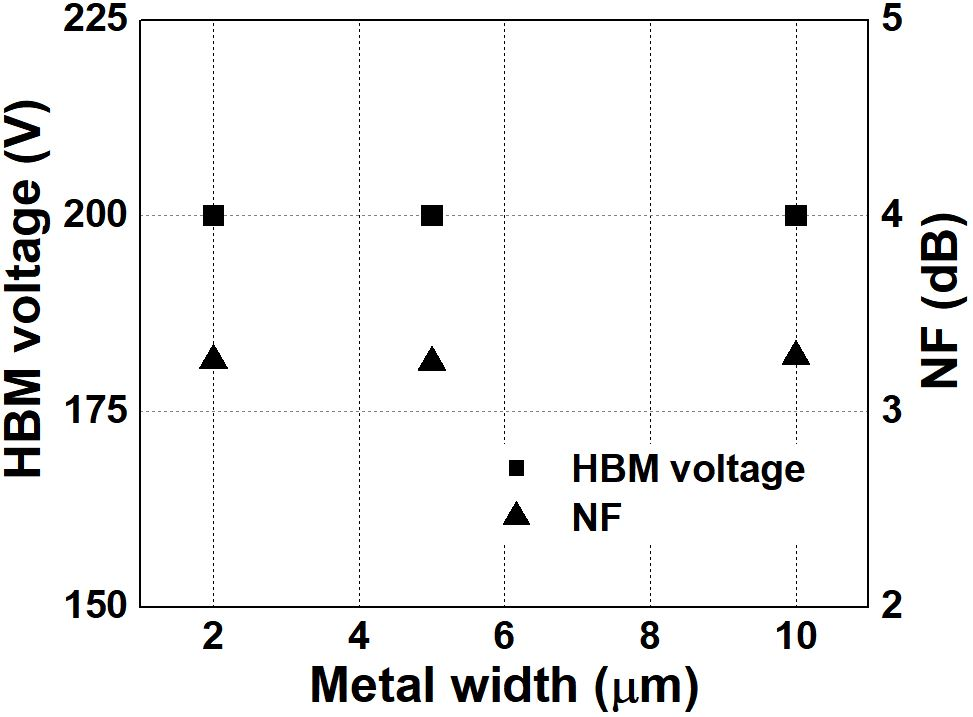Fig. 5. Simplified schematic of the proposed I/Q down-conversion mixer.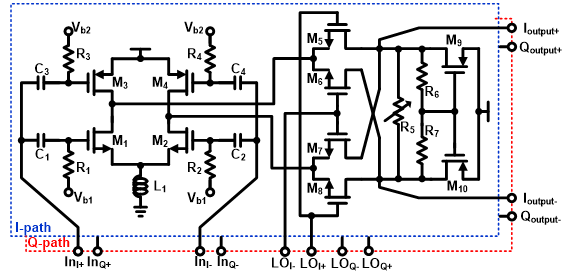Fig. 6. (a) Inverter transconductor and switching transistors from down-conversion mixer, (b) g$_{m}$$^{\prime}$$^{\prime}$ of small signal output current i$_{\mathrm{o}}$ of inverter transconductor versus the width of PMOS M$_{\mathrm{p1}}$ with a channel length of 65 nm.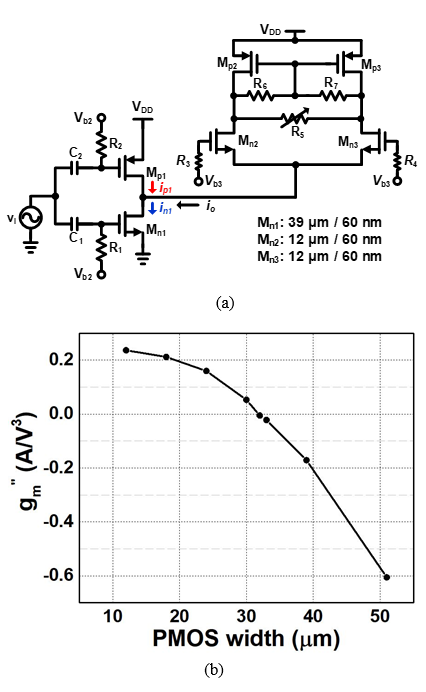Fig. 5 shows the simplified of the proposed I/Q down-conversion mixer. An inverter-type transconductor composed of NMOS and PMOS is used at the transconductance stage of the I/Q down-conversion mixer. The inverter-type transconductor has larger transconductance than a conventional NMOS counterpart at the same dc current consumption (8,9).

As shown in Fig. 6(a), if a small signal input voltage v$_{i}$ is applied to the inverter transconductor of the down-conversion mixer, the small signal output current I$_{o}$ can be expressed as

##### (4)
$\begin{array}{c} i_{o}=i_{n1}- i_{p1}=g_{m}v_{i}+g'_{m}v_{i}^{2}+g''_{m}v_{i}^{3}+\cdots \\ =\left(g_{m,n1}+g_{m,p1}\right)v_{i}+\left(g'_{m,n1}- g'_{m,p1}\right)v_{i}^{2}\\ +\left(g''_{m,n1}+g''_{m,p1}\right)v_{i}^{3}+\cdots \end{array}$,

where g$_{m}$$^{\prime} is the first derivative and g_{m}$$^{\prime}$$^{\prime} is the second derivative of the transconductance g_{m} with respect to the small signal input voltage v_{i}, respectively. Since the third-order nonlinearity of CMOS circuit is dominated by g_{m}$$^{\prime}$$^{\prime} nonlinearity (10), g_{m,n1}$$^{\prime}$$^{\prime} + g_{m,p1}$$^{\prime}$$^{\prime} should be zero to achieve high linearity. By adjusting the proper size and bias point of PMOS M_{\mathrm{p1}}, the g_{m,n1}$$^{\prime}$$^{\prime} term of NMOS M_{\mathrm{n1}} can be cancelled by the g_{m,p1}$$^{\prime}$$^{\prime} term of PMOS M_{\mathrm{p1}}. As shown in Fig. 6(b), when the width of PMOS M_{\mathrm{p1}} with a channel length of 65 nm is approximately 32 µm, the second derivative g_{m}$$^{\prime}$$^{\prime}$ of the transconductance g$_{m}$ with respect to the small signal input voltage v$_{i}$ is zero.

Fig. 7 shows the simulated output-referred third-order intercept point (OIP3) of the proposed down-conversion mixer according to the width of PMOS M$_{\mathrm{p1}}$ with a channel length of 65 nm. The largest simulated OIP3 result of the down-conversion mixer is obtained when the width of PMOS M$_{\mathrm{P1}}$ with a channel length of 65 nm is approximately 35 µm. The proposed I/Q down-conversion mixer improves more than 3 dB OIP3 performance in comparison to the conventional I/Q down-conversion mixer with an NMOS transconductor.

Fig. 7. Simulated OIP3 result of the proposed down-conversion mixer versus the width of PMOS M$_{\mathrm{p1}}$ with a channel length of 65 nm.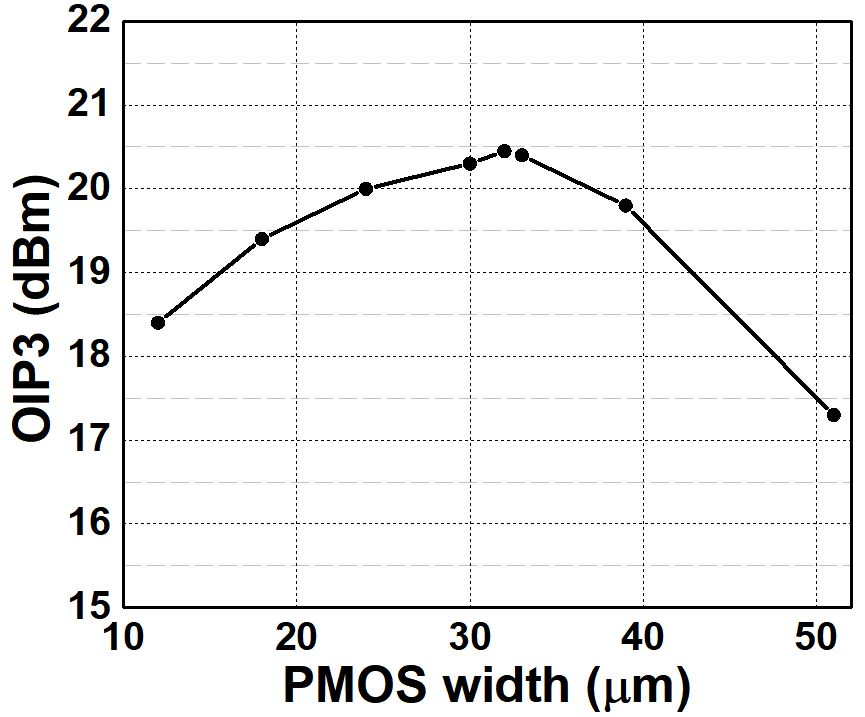Fig. 8. Simplified schematic of the I/Q LO generation circuit.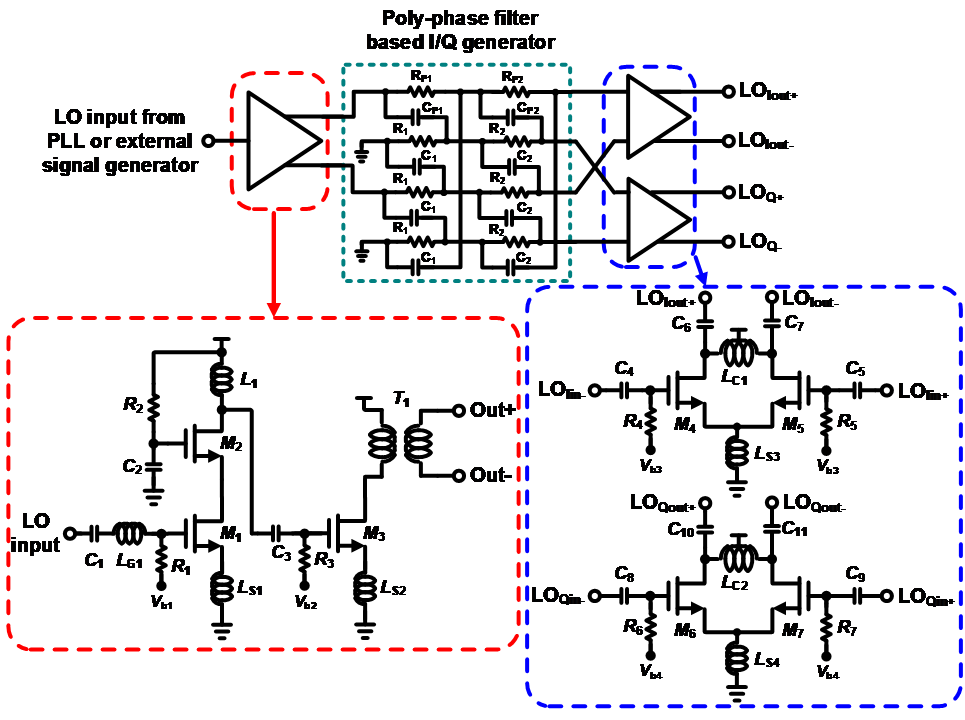Fig. 10. Measured RF input return loss of the RF front-end.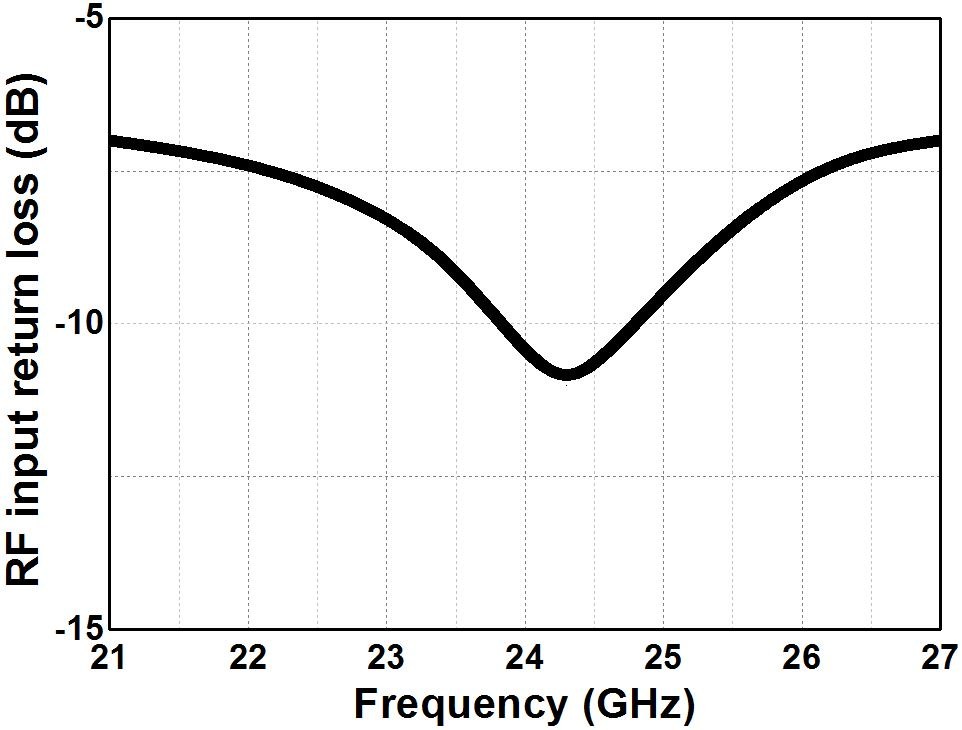The balancing accuracy between the I and Q paths is critical in achieving the targeted signal-to-noise ratio in the direct-conversion receiver. Especially, it is important to obtain balanced I/Q LO signals in the millimeter-wave band (11). Fig. 8 shows the simplified schematic of the I/Q LO generation circuits. The I/Q LO generation circuit consists of a single-ended cascode amplifier, a single-to-differential common-source amplifier, two-stage RC polyphase filter (PPF), and two differential common-source amplifiers. These amplifiers are used to compensate for the signal attenuation by two-stage PPF composed of passive components and to drive the switching pairs of the down-conversion mixer. The values of the resistance and capacitance of the two-stage PPF are destermined by considering the parasitic inductance value from equation in (12).

## III. Experimental Results

The proposed 24-GHz direct-conversion receiver front-end was implemented using a 65-nm CMOS process as part of an in-cabin radar system. Fig. 9 shows the chip photograph of the receiver front-end. The silicon area of the receiver front-end is 1250 ${\mathrm{\mu}}$m ${\times}$ 550 ${\mathrm{\mu}}$m excluding ESD protection circuits and PADs. It draws 21-mA from a 1.2-V supply voltage. For the measurement, an external signal generation instrument is used to provide the LO input signal. The measured return losses of the RF input and LO input are less than -10 dB in the frequency range from 24 GHz to 24.5 GHz.

Fig. 10 presents the measured RF input return loss of the RF front-end, which is less than -10 dB from 24 GHz to 24.5 GHz. Fig. 11 shows the measured conversion gain and phase mismatch between the I-path and Q-path at the IF output with an IF of 10 MHz of the RF front-end versus RF input frequency. The RF front-end shows a conversion gain of greater than 30 dB in the frequency range of 24 - 24.5 GHz. The gain and phase mismatch

between the I- and Q-paths at the IF output are less than 0.2 dB and 0.5$^{\circ}$ in the frequency range from 24 GHz to 24.5 GHz, respectively.

Fig. 11. Measured conversion gain and phase mismatch between I-path and Q-path at the IF output of the proposed RF front-end.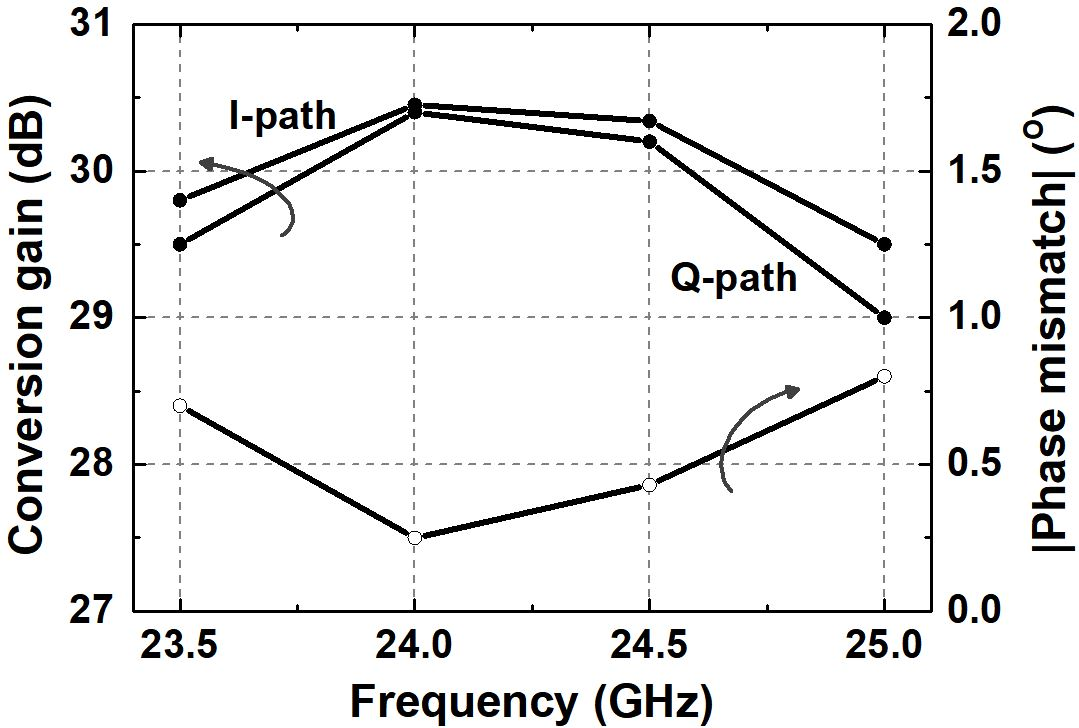Fig. 12. Measured IIP3 and DSB NF of the proposed RF front-end versus operating frequencies.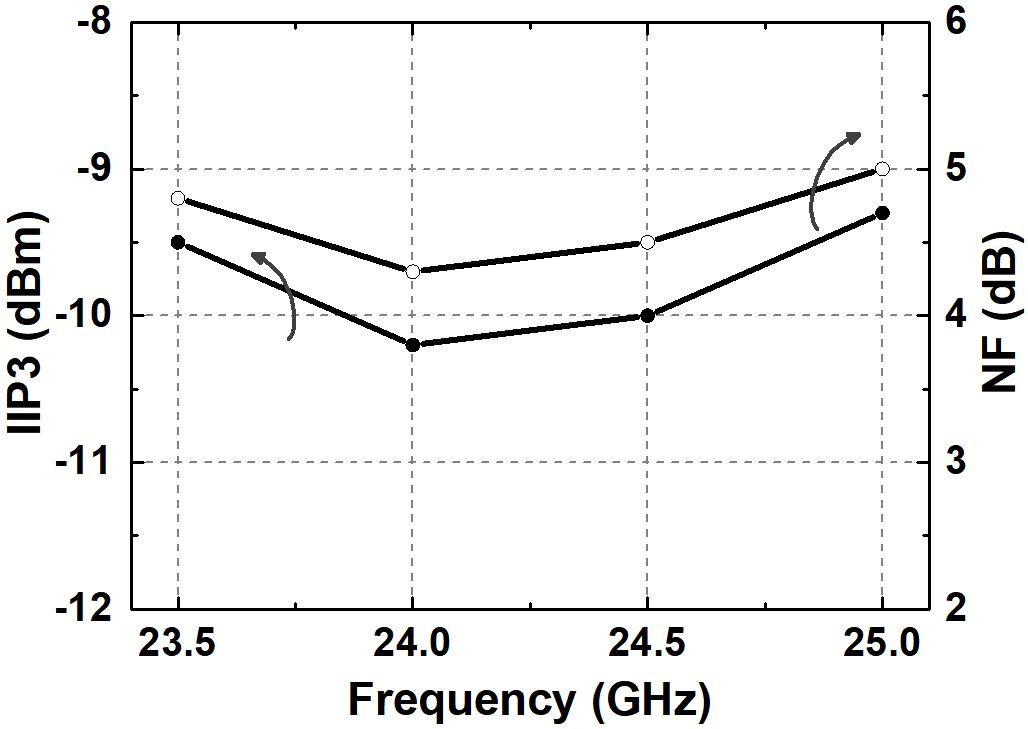Table 1. Summary and Comparison of Performance

 (8) (9) (10) This work Operating frequency (GHz) 24 25 24 24 Gain (dB) 31.5 15 36.7 30.4 OIP3 (dBm) 18.5 -4.4(1) 21.1 20.2 NF (dB) 6.7 9.5 6.1 4.5 P1dB (dBm) -24 -29 -29.7 -23 Power consumption (mW) 78 @ 1.2V (LNA + I/Q mixer + VCO & dividers) 20 @ 1.2V (LNA + I/Q mixer + LO generator) 66 @ 1.5V (LNA + I/Q mixer + LO generator) 25.2 @ 1.2V (LNA: 8 mA + I/Q mixer: 4 mA + LO generator: 9 mA) Technology 65 nm CMOS 130 nm CMOS 130 nm CMOS 65 nm CMOS Area 0.24 $mm^{2}$ 0.84 $mm^{2}$ 1.76 $mm^{2}$ 0.69 $mm^{2}$ F.O.M(2) 0.15 0.05 0.19 1.35

$^{(1)}$ OIP3 ${\approx}$ Gain + IIP3 (= P$_{\mathrm{1dB}}$ + 9.6dB),

$^{(2)}$ $^{\mathrm{F.O.M.=\frac{Gain[abs]\cdot IIP3[mW]}{P_{DC}[mW]}\cdot \frac{1}{(NF- 1)[abs]}\cdot f[GHz]}}$

Fig. 12 shows the measured IIP3 and double-side band (DSB) noise figure (NF) results of the proposed RF front-end, respectively. Two-tone spacing is 2 MHz for the linearity test. The RF front-end has an IIP3 of greater than -10 dBm over the entire operating frequency bands. The RF front-end has a DSB NF of less than 4.5 dB over the entire operating frequency bands.

Table 1 summarized and compares the performances of the proposed RF front-end with those of other published mm-wave RF front-ends. The proposed RF front-end has good figure of merit (FOM) compared with the recently published millimeter-wave RF front-ends.

## IV. CONCLUSIONS

A 24-GHz direct-conversion receiver front-end was presented for in-cabin applications. The proposed RF receiver is composed of a low-noise amplifier, an I/Q down-conversion mixer, and an I/Q LO generator circuit. An inverter transconductor with third-order nonlinearity cancellation is applied to the I/Q down-conversion mixer to improve the linearity of the I/Q down-conversion mixer. By adopting a linear I/Q down-conversion mixer and a balanced I/Q LO generator, the 24 GHz receiver front-end obtains high linearity and good I/Q balancing performance. The mm-wave receiver front-end can be utilized to in-cabin radar systems that can monitor driver’s vital signs such as heart rate and respiration.

### ACKNOWLEDGMENTS

This work was supported by the National Research Foundation of Korea (NRF) grant funded by the Ministry of Science and ICT (NRF-2019R1A2C1090935).

This research was supported by National R&D Program through the National Research Foundation of Korea (NRF) funded by Ministry of Science and ICT (2020M3H2A1078045).

This research was supported by Korea Institute for Advancement of Technology (KIAT) grant funded by the Korea Government (MOTIE) (P0012451, The Competency Development Program for Industry Specialist).

The CAD tools were supported by IDEC.

### REFERENCES

1
Droitcour A., Lubecke V., Lin J., Boric-Lubecke O., May 2001, A microwave radio for doppler radar sensing of vital signs, In Proceedings of the 2001 IEEE MTT-S International Microwave Symposium Digest Phoenix AZ US, pp. 175-1782
Xiao Y., Lin J., Boric-Lubecke O., Lubecke M., May 2006, Frequency-tuning technique for remote detection of heartbeat and respiration using low-power double-sideband transmission in the ka-band, IEEE Trans Microw Theory Tech, Vol. 54, No. 5, pp. 2023-20323
Park J.-H., Jeong Y.-J., Lee G.-E., Oh J.-T., Yang J.-R., May 2019, 915-MHz continuous-wave doppler radar sensor for detection of vital signs, Electronics, Vol. 8, No. 5, pp. 8554
Andersen N., Granhaug K., Dec 2017, A 118-mW pulse-based radar SoC in 55-nm CMOS for non-contact human vital signs detection, IEEE J. Solid State Circuits, Vol. 52, No. 12, pp. 3421-34335
Nguyen T. N., Cha H.-K., Feb 2021, Design of a low-power 10-bit 250-kS/s SAR ADC for neural recording applications, IEIE Trans Smart Process. Comput, Vol. 10, No. 1, pp. 67-736
Nam I., Choi K., Lee J., Cha H.-K., Seo B.-I., Kwon K., Lee K., Apr 2007, A 2.4-GHz low-power low-IF receiver and direct-conversion transmitter in 0.18~µm CMOS for IEEE 802.15.4 WPAN applications, IEEE Trans. Microw. Theory Tech, Vol. 55, No. 4, pp. 682-6897
Khan A., Electro-static Discharge(ESD) Tutorial, Cypress Semiconductor Corp, https://www.cypress.com/documentation/white-papers/electro-static-discharge-esd-tutorial.8
Karanicolas A., Dec 1996, A 2.7 V 900 MHz CMOS LNA and mixer, IEEE J. Solid State Circuits, Vol. 31, No. 12, pp. 1939-19449
Choi C., Kwon K., Nam I., 2016, A 370 µW CMOS MedRadio receiver front-end with inverter-based complementary switching mixer, IEEE Microw. Wirel. Compon. Lett, Vol. 26, pp. 73-7510
Nam I., Kim B., Lee K., May 2005, CMOS RF amplifier and mixer circuits utilizing complementary charac-teristics of parallel combined NMOS and PMOS devices, IEEE Trans. Microw. Theory Tech., Vol. 53, No. 5, pp. 1662-167111
Shin B., Kim C., Lee O., Park S., Noh S., Nam I., Apr 2018, A 24 GHz receiver front-end with improved I/Q balanced LO generator in 65-nm CMOS for surveillance radar systems, Journal of Semicon-ductor Technology and Science, Vol. 18, No. 4, pp. 475-47912
Lee S., Jeon Y., Park G., Myung J., Lee S., Lee O., Moon H., Nam I., Dec 2020, A 24-GHz RF transmitter in 65-nm CMOS for in-Cabin radar applications, Electronics, Vol. 9, No. 12, pp. 2005## Author

received the B.S. degree in electronic engineering from Pukyong National University, Busan, Korea, in 2017, and the M.S. degree in electrical engineering at Pusan National University, Busan, Korea.

Her main interests are CMOS RF/ mmWave/analog circuits for wireless communications.

received the B.S. degree in electrical engineering from Pusan National University, Busan, Korea, in 2020 and is currently working toward the M.S. degree in electrical and electronics engineering at Pusan National University, Busan, Korea.

Her main interests are CMOS RF/mmWave/analog circuits for wireless communications.

received the B.S. degree in Electronic Engineering from Dong-A University, Busan, Korea, in 2019, and he is currently pursuing the combined M.S/Ph.D. degree in Electrical Engineering at Pusan National University, Busan, Korea.

His main interests are CMOS RF/mmWave/ PMIC/analog circuits for wireless communications.

received the B.S degree in Information and Communi-cation Engineering from Kyungsung University, Busan, Korea, in 2019 and currently working toward the M.S. degree in Electrical Engineering at Pusan National University, Busan, Korea.

His main interests are CMOS RF/mmWave/ analog circuits for wireless communications.

received the B.S. degree in Electronic Engineering from Gyeongsang National Univer-sity, Gyeongsangnam-do, Korea, in 2019, and the M.S degrees in Electrical Engineering from Pusan National University, Busan, Korea, in 2021, and is currently working toward the Ph.D. degree in Electrical and Electronic Engineering at Pusan National University, Busan, Korea.

His current research interests are CMOS RF/PMIC/analog circuits for wireless communications.

received the B.S. degree in electrical engineering from Sungkyunkwan University, Korea, in 2001, the M.S. degree in electrical engineering from the KAIST, Korea, in 2005, and the Ph.D. degree in electrical and computer engineering from the Georgia Institute of Technology, USA, in 2009.

Upon completion of the doctoral degree, he joined Qualcomm Inc., USA, as a Senior Engineer, where he was involved in the development of transmitters and integrated passive circuits on mobile applications.

He is currently a faculty member with the Department of Electrical Engineering, Pusan National University, Korea.

His research interests include high-frequency integrated circuits and system design for wireless communications and biomedical applications.

received a B.S. degree in radio science and engi-neering from Hanyang University, Korea, in 1997 and M.S. and Ph.D. degrees in EECS from KAIST, Korea, in 1999 and 2004, respectively.

In 2004, he joined Samsung Electronics, Gyeonggi, Korea, as a senior engineer, designed multi-band multi-mode RF transceiver ICs for a cellular phone, and developed a receiver IC for a wireless connectivity system such as GPS and FM. In 2012, he joined the school of Electronic and Electric Engineering, Daegu University, Gyeongsan, Korea, as an associate professor.

His research interests including CMOS RF/mmWave/analog integrated circuits and systems for wireless communications such as WSNs and 5G cellular systems.

received the B.S. degree in electronics engineering from Yonsei University, Korea, in 1999, and the M.S. and Ph.D. degrees in electrical engineering and computer sciences from the KAIST, Korea, in 2001 and 2005, respectively.

From 2005 to 2007, he was a Senior Engineer with Samsung Electronics, Gyeonggi, Korea, where he was involved in the development of mobile digital TV tuner IC.

In 2007, he joined the School of Electrical Engineering, Pusan National University, Busan, Korea, and is now a Professor.